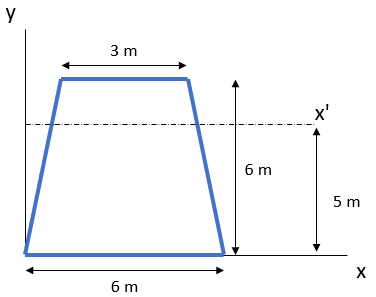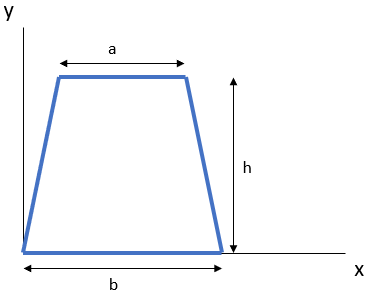## Moment of Inertia

Calculate the moment of inertia of the trapezoid area about the x' axis. Assume the centroid axis line is 2.6 m away from the x-axis.Hint
Parallel Axis Theorem: The moment of inertia of an area about any axis is defined as the moment of inertia of the area about a parallel centroidal axis plus a term equal to the area multiplied by the square of the perpendicular distance $$d$$ from the centroidal axis to the axis in question.
$$I_{x'}=I_{xc}+d_{y}^{2}A$$$where $$A$$ is the area of the trapezoid: Hint 2 First, find the moment of inertia about the centroid axis: $$I_{xc}=\frac{h^3(a^2+4ab+b^2)}{36(a+b)}$$$First, let's find the moment of inertia about the centroid axis:
$$I_{xc}=\frac{h^3(a^2+4ab+b^2)}{36(a+b)}$$$$$I_{xc}=\frac{6^3(3^2+4(3)(6)+(6)^2)}{36(3+6)}=\frac{216(117)}{324}=78$$$
Parallel Axis Theorem: The moment of inertia of an area about any axis is defined as the moment of inertia of the area about a parallel centroidal axis plus a term equal to the area multiplied by the square of the perpendicular distance $$d$$ from the centroidal axis to the axis in question.
$$I_{x'}=I_{xc}+d_{y}^{2}A$$$where $$A$$ is the area of the trapezoid: $$A=\frac{h(a+b)}{2}=\frac{6(3+6)}{2}=27$$$
Finally:
$$I_{x'}=78+(5-2.6)^{2}(27)=233.52\:m^4$$$$$233.52\:m^4$$$